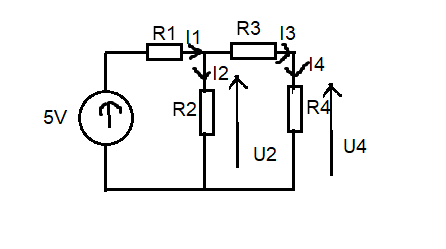# [beginner question] Calculate currents in simple circuit (Ohm's law)

Hi,

how to calculate I1, I2, I3, I4 in this circuit?

R1 = 500 Ohms
R2 = 600 Ohms
R3 = 4000 Ohms
R4 = 200 Ohms

U2 = 2,5V
U4 = 0,1V